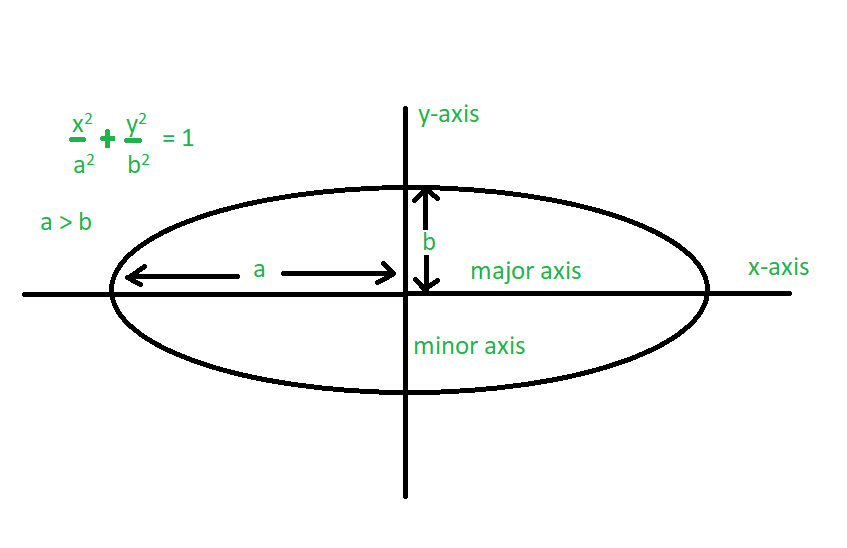# Perimeter of an Ellipse

An ellipse is described as a curve on a plane that surrounds two focal points such that the sum of the distances to the two focal points is constant for every point on the curve. Ellipse has two types of axis – Major Axis and Minor Axis. The longest chord of the ellipse is the major axis. The perpendicular chord to the major axis is the minor axis which bisects the major axis at the center.

Given the lengths of minor and major axis of an ellipse, the task is to find the perimeter of the Ellipse.Examples:

Input: a = 3, b = 2
Output: 16.0109

Input: a = 9, b = 5
Output: 45.7191

## Recommended: Please try your approach on {IDE} first, before moving on to the solution.

Perimeter of an ellipse is:

Perimeter : 2π * sqrt( (a2 + b2) / 2 )

Where a and b are semi-major axis and semi-minor axis respectively.

Below is the implementation of the above approach:

## C++

 `// C++ program to find perimeter of an Ellipse  ` `#include   ` `using` `namespace` `std;  ` ` `  `// Function to find the perimeter of an Ellipse  ` `void` `Perimeter(``int` `a, ``int` `b)  ` `{  ` `    ``float` `perimeter;  ` ` `  `    ``// Compute perimeter  ` `    ``perimeter = 2 * 3.14 * ``sqrt``((a * a + b * b) / (2 * 1.0));  ` ` `  `    ``cout << perimeter;  ` `}  ` ` `  `// Driver code  ` `int` `main()  ` `{  ` `    ``int` `a = 3, b = 2;  ` ` `  `    ``// Function call  ` `    ``Perimeter(a, b);  ` ` `  `    ``return` `0;  ` `}  `

## Java

 `// Java program to find perimeter of  ` `// an Ellipse.  ` `import` `java.lang.Math; ` `class` `GFG1{  ` `   `  `    ``// Function to find perimeter of an  ` `    ``// ellipse.  ` `    ``static` `void` `Perimeter( ``double` `a, ``double` `b)  ` `    ``{  ` `        ``double` `Perimeter;  ` `           `  `        ``// formula to find the Perimeter ` `        ``// of an Ellipse.  ` `        ``Perimeter = (``double``)``2` `* ``3.14` `* Math.sqrt((a * a + b * b) / (``2` `* ``1.0``)) ;  ` `           `  `        ``// Display the result  ` `        ``System.out.println(``"Perimeter: "` `+ Perimeter); ` `    ``}  ` `       `  `    ``// Driver code  ` `    ``public` `static` `void` `main (String[] args)  ` `    ``{  ` `        ``double` `a = ``3``, b = ``2``;  ` `           `  `        ``Perimeter(a , b); ` `    ``}  ` `}  `

## Python3

 `# Python3 program to find perimeter ` `# of an Ellipse ` `from` `math ``import` `sqrt ` ` `  `# Function to find the perimeter  ` `# of an Ellipse ` `def` `Perimeter(a, b): ` `    ``permeter ``=` `0` ` `  `    ``# Compute perimeter ` `    ``permeter ``=` `(``2` `*` `3.14` `*`  `                ``sqrt((a ``*` `a ``+` `b ``*` `b) ``/`  `                           ``(``2` `*` `1.0``))); ` ` `  `    ``print``(permeter) ` ` `  `# Driver code ` `a ``=` `3` `b ``=` `2` ` `  `# Function call ` `Perimeter(a, b) ` ` `  `# This code is contributed  ` `# by Mohit Kumar `

## C#

 `// C# program to find perimeter of  ` `// an Ellipse.  ` `using` `System; ` `     `  `class` `GFG1 ` `{  ` `     `  `    ``// Function to find perimeter of an  ` `    ``// ellipse.  ` `    ``static` `void` `Perimeter(``double` `a, ``double` `b)  ` `    ``{  ` `        ``double` `Perimeter;  ` `             `  `        ``// formula to find the Perimeter ` `        ``// of an Ellipse.  ` `        ``Perimeter = (``double``)2 * 3.14 *  ` `                     ``Math.Sqrt((a * a + b * b) / (2 * 1.0));  ` `             `  `        ``// Display the result  ` `        ``Console.WriteLine(``"Perimeter: "` `+ Perimeter); ` `    ``}  ` `         `  `    ``// Driver code  ` `    ``public` `static` `void` `Main (String[] args)  ` `    ``{  ` `        ``double` `a = 3, b = 2;  ` `             `  `        ``Perimeter(a , b); ` `    ``}  ` `} ` ` `  `// This code is contributed by Princi Singh `

Output :

``` 16.010921272681344
```

Attention reader! Don’t stop learning now. Get hold of all the important DSA concepts with the DSA Self Paced Course at a student-friendly price and become industry ready.

My Personal Notes arrow_drop_upCheck out this Author's contributed articles.

If you like GeeksforGeeks and would like to contribute, you can also write an article using contribute.geeksforgeeks.org or mail your article to contribute@geeksforgeeks.org. See your article appearing on the GeeksforGeeks main page and help other Geeks.

Please Improve this article if you find anything incorrect by clicking on the "Improve Article" button below.

Article Tags :
Practice Tags :

Be the First to upvote.

Please write to us at contribute@geeksforgeeks.org to report any issue with the above content.